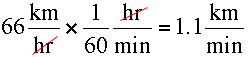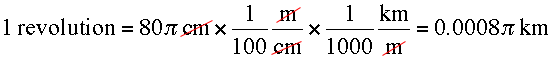# The wheels of a car are of diameter 80 cm each. How many complete revolutions does each wheel make in 10 minutes when the car is traveling at a speed of 66 km per hour? please write in a simple method?

Given: speed of the car = 66 km/h

∴ Distance travelled by the car in 1 hour or 60 minutes = 66 km/h

⇒ Distance travelled by the car in 10 minutes == 11 km = 11 × 1000 m

= 11 × 1000 × 100 cm

Diameter of car wheel = 80 cm

∴ radius of car wheel =∴ Circumference of the wheel = 2πrwe know that, circumference of the wheel = Distance travelled by the car when its wheels take one complete revolution∴ Number of revolutions made by wheels in 10 minutes= 4375 revolutions

• 138

66 km per hour isThe car travels at this speed for 10 minutes and hence it travels 11 km.

The diameter of the wheel is 80 cm so its circumference measures 80 π cm. thusSo how many revolutions are there in 11 km?

• -20

c.s.a of the wheel=2* 227*40

speed of the car in 1 min=6660

speed of the car in 10 min = 6660*10

speed of the car in 10 mins =11*1000*100=11000 *100

no.of revolutions=1100000*7/2*22*40

=4375

• 22

DIAMETER OF WHEEL = 80 cm  ; therefore radius = 40cm

speed of car = 66 km/hr = 66 * 1000 /3600 m/s = 66*1000*100/3600 cm/s

therefore distance covered by wheel in 1 sec = 66*1000*100/3600 cm

therefore distance covered by wheel in 10minutes (10 * 60) = 66*1000*100*10*60/3600 = 11* 10 5 cm

distance covered in one revolution =circumference of wheel = 2 * 22/7 * 40 cm

therefore no of revolution in 1 cm = 7/2*22*40

therefore no of revolution in 10 min =therefore no of revolution in 11 * 10 5cm = 7/2*22*40 * 11 *10 5 = 4375

• 6
What are you looking for?